#### How to Do to the Power of in Excel

In this Excel lesson, you will learn how to use exponents.

## Definition

Exponent means how many times the number will be multiplied by itself. For example, 103 (10 to the 3rd power) equals 10 * 10 * 10, which is 1,000.

The power of exponent in Excel is a caret symbol (SHIFT + 6 keyboard shortcut), which is ^. So you will write 10 to the 3rd power in Excel by 10^3.

## Calculations

### Using Caret

To type exponents in Excel, just use caret.

In the cell, you can just write =10^3.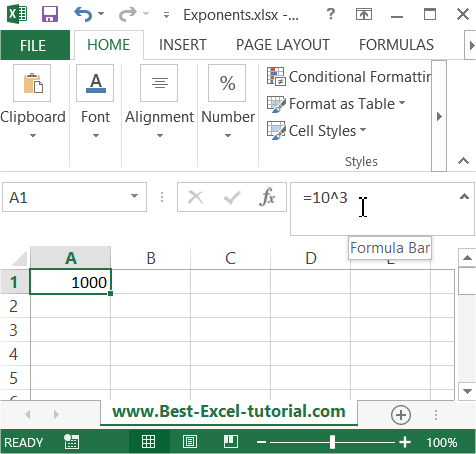Of course, a cell address, such as =10^A2, is an option.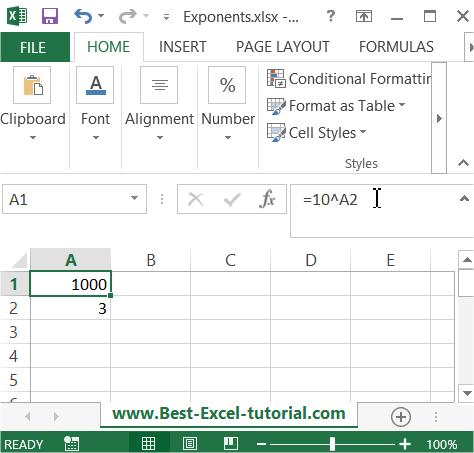Also, =A1^3 is possible.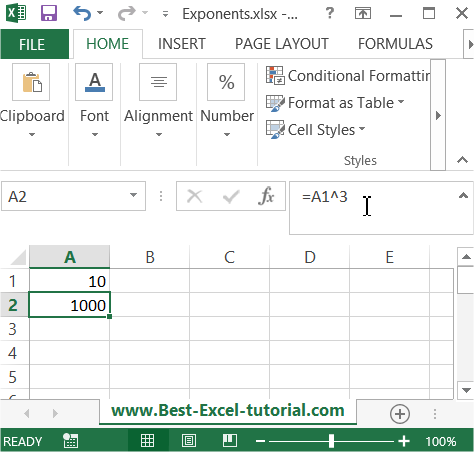And =A1^A2 as well.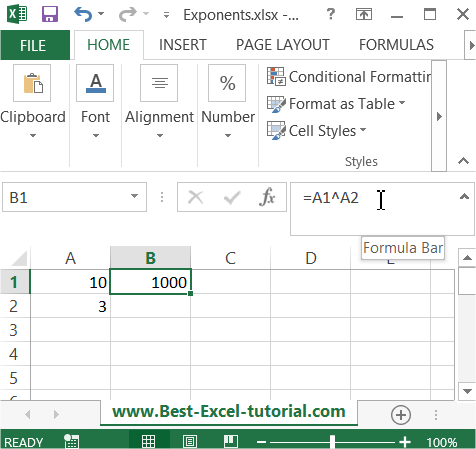### Using POWER function

Excel offers the POWER function for exponent calculations. Write =POWER(10,3) to calculate our example.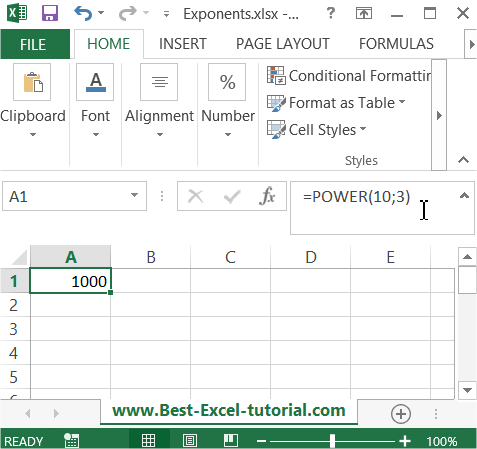You can use the caret or POWER function. Choose the one that you prefer. Maths is much easier with Excel. The ^ operator and the POWER function both perform the same calculation, but the POWER function provides more versatility and control over the calculation, as it allows you to enter the arguments directly into the formula, rather than using the ^ operator.

## Negative Exponents

Excel can handle negative exponents as well. For instance, to calculate 10 to the power of -2 (which is equivalent to 1 divided by 10 squared), you can use the formula “=10^-2”.

### Fractional Exponents

You can use fractional exponents to calculate roots of numbers. For example, to calculate the square root of a number, you can use the formula “=A1^(1/2)”.

See also  How to create IN and OUT Inventory System in Excel?

## Exponents in Functions

Incorporate exponents within various Excel functions. For instance, you can use exponents in financial functions like Future Value (FV) and Present Value (PV) to model the time value of money.

Excel provides both basic and advanced tools for working with exponents, making it a versatile tool for a wide range of mathematical and scientific applications. Whether you’re a student, a professional, or a researcher, Excel can help you handle exponents efficiently.